Next: 5.1.1 Cartesian Linear Transformation Up: 5. GMT Coordinate Transformations Previous: 5. GMT Coordinate Transformations   Contents   Index

# 5.1 Cartesian Transformations

GMT Cartesian coordinate transformations come in three flavors:

• Linear coordinate transformation
• Log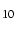coordinatetransformation
• Power (exponential) coordinate transformation

These transformations convert input coordinates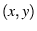to locations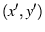on a plot. There is no coupling between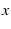and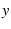(i.e.,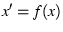and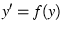); it is a one-dimensional projection. Hence, we may use separate transformations for the-,-, and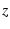-axes. Below, we will use the expression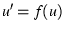, where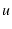is eitheror(orfor 3-D plots). The coefficients in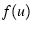depend on the desired plot size (or scale), the chosendomain, and the nature of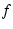itself.

Two subsets of linear will be discussed separately; these are a polar (cylindrical) projection and a linear projection applied to geographic coordinates (with a 360 degree periodicity in the-coordinate). We will show examples of all of these projections using dummy data sets created with gmtmath, a ``Reverse Polish Notation'' (RPN) calculator that operates on or creates table data:

```gmtmath -T0/100/1  T SQRT = sqrt.d
gmtmath -T0/100/10 T SQRT = sqrt.d10
```

SubsectionsNext: 5.1.1 Cartesian Linear Transformation Up: 5. GMT Coordinate Transformations Previous: 5. GMT Coordinate Transformations   Contents   Index
Paul Wessel 2004-10-01